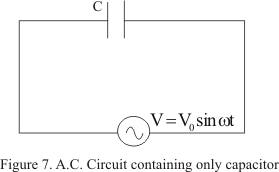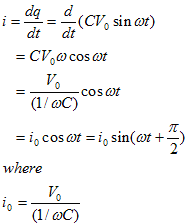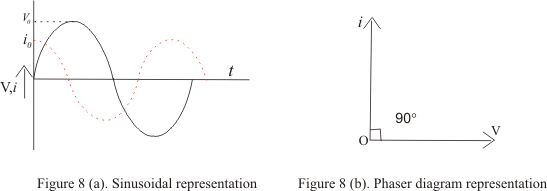# Alternating current(AC) through Capacitor

## AC through pure capacitor

• Figure given below shows circuit containing alternating voltage source
V=V0sinωt
connected to a capacitor of capacitance C• Suppose at any time t,q be the charge on the capacitor and i be the current in the circuit
• Since there is no resistance in the circuit, so the instantaneous potential drop q/C across the capacitor must be equal to applied alternating voltage
so
q/C=V0sinωt
• Since i=dq/dt is the instantaneous current in the circuit sois the peak value of current
• Comparing equation (13) with V=V0sinωt ,we see that in a perfect capacitor current leads emf by a phase angle of π/2
• This phase relationship is graphically shown below in the figure• Again comparing peak value of current with ohm's law ,we find that quantity 1/ωC has the dimension of the resistance
• Thus the quantity
XC=1/ωC =1/2πfC ---(14)
is known as capacitive reactance
• From equation (14) we see that capacitive reactance decreases with increasing frequency of current and in infinite for direct current for which frequency f=0